Math Trivia
Exponents
Order of Operations
Random Riddles
Percent
Misc.
100

What is the distance a number is from zero on the number line?

Absolute Value

100

24

16

100

Simplify the following expression: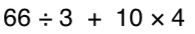100

-4 + -5

9100

What has to be broken before you can use it?

an egg

100

What is 3/4 as a percent?

75%

100

The family of shapes that each have four sides are called?

200

If you are filling a swimming pool with water, will you be solving for volume or surface area?

Volume

200

What is 9^3 (exponent)

729

200

Simplify the following expression: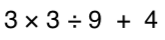200

14 + -12

2

https://www.khanacademy.org/math/arithmetic/arith-review-negative-numbers/arith-review-add-negatives-intro/v/adding-negative-numbers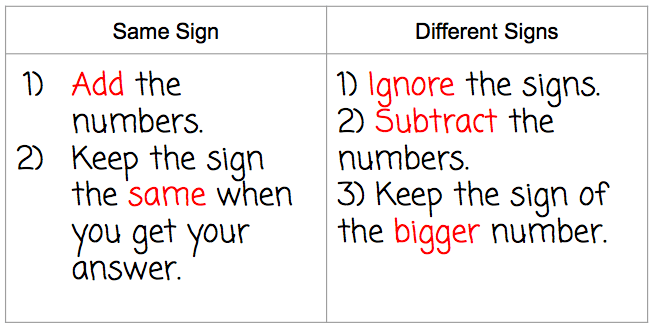200

What begins with T, finishes with T, and has T in it?

a teapot

200

What is 4/5 as a percent?

80%

200
In Translation, a movement to the left is represented by what kind of number?

A negative number

300

What do you call the probability where you are using the data from an experiment to find the probability of an event happening? Experimental or Theoretical

Experimental

300

what is 25

32

300

3 + 42 * (3 + 2)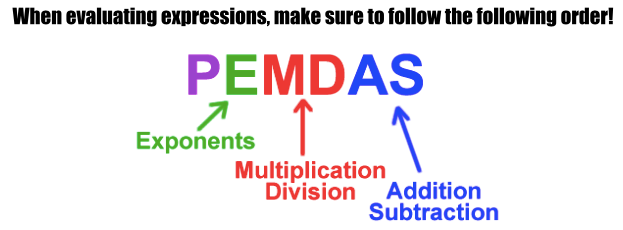300

-10 + 15

5300

What goes up but never comes back down?

300

95% is what as a fraction in simplest form?

19/20

300

Sam is 3 years older that his sister Pam. If the sum of their ages is 17, then how old is Sam?

10 years old

400

A trapezoid has 2 sets of parallel sides.  True or False

False

400

What is the value of:

84

4,096

400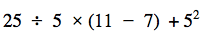400

-2 + -3 + 5

0

https://www.khanacademy.org/math/arithmetic/arith-review-negative-numbers/arith-review-add-negatives-intro/v/adding-negative-numbers400

No matter what, what can you always count on?

400

60% is what as a decimal?

0.60

400

The number in front of a variable is called this.

A Coefficient

500

If an ordered pair is reflected over the y-axis, what coordinate will change to the opposite.

x-coordinate

500500

6 + (-2 + -5)

-1

500

What is full of holes but still holds water?

a sponge

500

What is 1/3 as a percent?

33.3%

500

WHAT AM I?

From the centre I start my run, but the arc stops my fun. Size is determined when I say how wide even though I'm only half the divide.